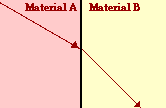# Ray Optics: Refraction and Lenses

## Refraction and Lenses: Audio Guided Solution

#### Problem 9:A light ray is traveling through crown glass (n = 1.52) and approaching the boundary with water (n = 1.33) as shown in the diagram at the right.
a. Use a protractor to measure the angle of incidence of the light ray in the crown glass.
b. Calculate the angle of refraction of the light ray as it enters into the water.

## Habits of an Effective Problem Solver

• Read the problem carefully and develop a mental picture of the physical situation. If necessary, sketch a simple diagram of the physical situation to help you visualize it.
• Identify the known and unknown quantities and record them in an organized manner. Equate given values to the symbols used to represent the corresponding quantity - e.g., do = 24,8 cm; di = 16.7 cm; f = ???.
• Use physics formulas and conceptual reasoning to plot a strategy for solving for the unknown quantity.
• Identify the appropriate formula(s) to use.
• Perform substitutions and algebraic manipulations in order to solve for the unknown quantity.